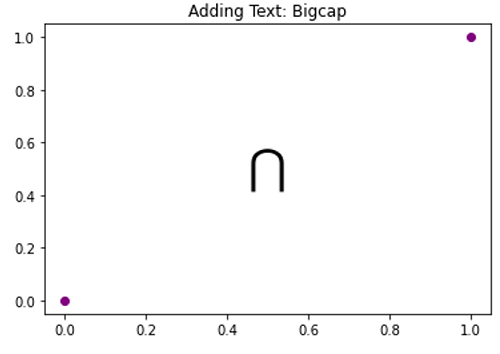# Bigcap Symbol in Python Plotting

Here, we are going to learn about the bigcap symbol in Python plotting and its implementation.
Submitted by Anuj Singh, on August 31, 2020

Bigcap is a different symbol used in set theory and python has provided us to use it while plotting. Following example shows its implementation.

```plt.text(0.45, 0.45, r'\$\bigcap\$')
```

Illustrations:## Python code for bigcap symbol in plotting

```import numpy as np
import matplotlib.pyplot as plt

# Bigcap
# In text
plt.figure()
plt.plot([0,1],[0,1], 'o', color=  'purple')
plt.text(0.45, 0.45, r'\$\bigcap\$', fontsize=25, )
plt.show()
```

Output:

```Output is as Figure
```